## Wednesday, August 24, 2016

### Optimizing node rotations with `cophylo` under different conditions

The function `cophylo` creates an object of class `"cophylo"` and by default will perform node rotations in which the “matching” of tip nodes (either exactly, by tip label, or through the association matrix, `assoc`) is optimized. Matching is defined as the sum of squared differences between the height of the corresponding tip labels in each of the plotted trees. (It can also be defined differently - such as by the sum of the absolute values of the differences.)

The algorithm I employed is to first traverse tree 1 from root-to-tips (i.e., pre-order) and rotate each node, accepting node rotations that improve matching to tree 2. Next, the same procedure is performed on tree 2. If desired, both pre- and post-order traversals can be performed on each tree.

I do not have a proof that this algorithm is guaranteed to optimize the matching by my criterion (or any) between trees; however it is nonetheless my empirical observation that if a precise match exists, the algorithm will find it.

Here is a demonstration using a simulated 80 taxon tree in which I will first randomly rotate nodes 200 times using `rotateNodes`, next show how tangled this makes an unrotated `"cophylo"` plot look, then, finally, optimize the node rotations of the two trees and show that the algorithm results in perfect matching:

``````library(phytools)
## simulate trees
t1<-t2<-rtree(n=80)
## rotate nodes of t2 randomly
for(i in 1:200)
t2<-rotateNodes(t2,sample(1:t2\$Nnode+Ntip(t2),1))
## tangled "cophylo" plot
tangled<-cophylo(t1,t2,rotate=FALSE)
plot(tangled,fsize=0.5)
``````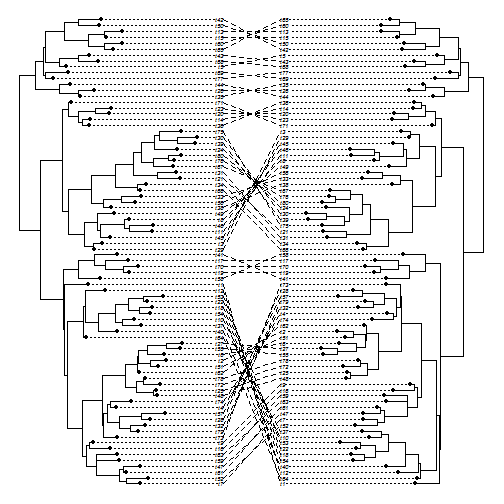``````## untangled "cophylo" plot
untangled<-cophylo(t1,t2,rotate=TRUE,print=TRUE)
``````
``````## Rotating nodes to optimize matching...
``````
``````## objective: 13202
## objective: 12012
## objective: 4362
...
...
## objective: 18
## objective: 2
## objective: 0
``````
``````## Done.
``````
``````plot(untangled,fsize=0.5)
``````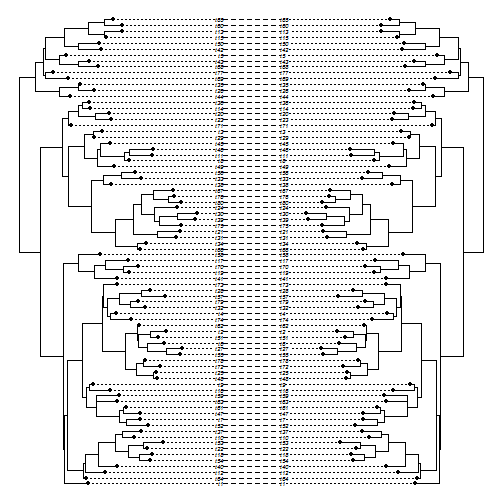Both input trees in the previous example are perfectly bifurcating. The method also has an option that works for multifurcating trees, `rotate.multi`, in which essentially we try all possible ordering of the daughter edges of each multifurcation. Note that this is will result in a prohibitively large number of trees to evaluate for the case of high order multifurcations. For instance, a multifurcation with 7 descendant daughter edges will have 7! = 5040 possible orderings.

In addition, we may need to perform both pre- and post-order tree traversal to optimize the matching. Here I will set a bunch of edges to zero in two identical trees - then perform random node rotations on one of them - then collapse all the zero-length edges to multifurcations in each tree. Finally, I will try to match both trees with `rotate.multi=TRUE` and `methods=c("pre","post")`:

``````ee<-which(t1\$edge[,2]>Ntip(t1))
t3<-t1
t3\$edge.length[sample(ee,20)]<-0
t4<-t3
for(i in 1:200)
t4<-rotateNodes(t4,sample(1:t4\$Nnode+Ntip(t4),1))
t3<-di2multi(t3)
t4<-di2multi(t4)
## with rotate.multi=FALSE
wo.multi<-cophylo(t3,t4,print=TRUE)
``````
``````## Rotating nodes to optimize matching...
``````
``````## objective: 20854
...
...
## objective: 3958
``````
``````## Done.
``````
``````plot(wo.multi,fsize=0.5)
``````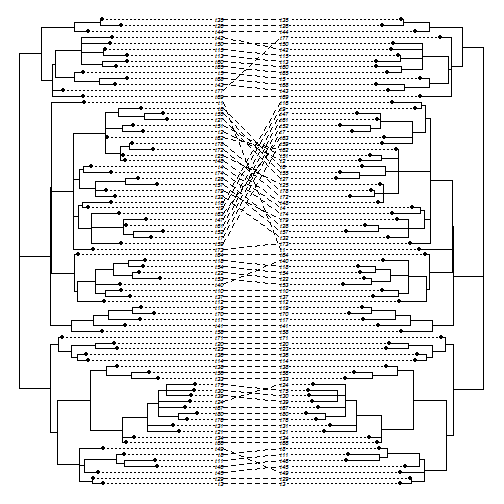``````## with rotate.multi=TRUE
w.multi<-cophylo(t3,t4,rotate.multi=TRUE,
methods=c("pre","post"),print=TRUE)
``````
``````## Rotating nodes to optimize matching...
``````
``````## objective: 89494
## objective: 82494
## objective: 82494
## objective: 82482
...
...
## objective: 1232
## objective: 882
## objective: 0
## objective: 0
``````
``````## Done.
``````
``````plot(w.multi,fsize=0.5)
``````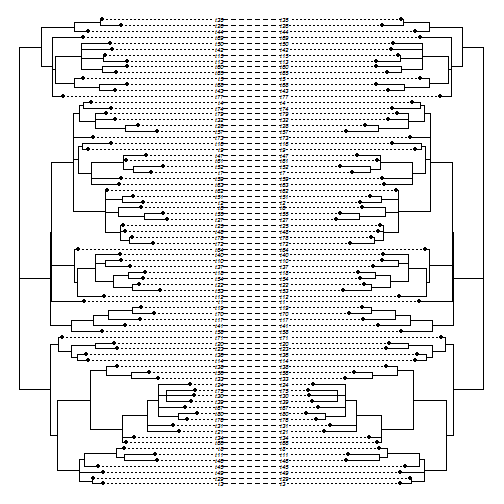In addition, I thought it might be worthwhile to demonstrate that the methods work even for quite large trees. For instance:

``````t1<-t2<-rtree(n=1000)
for(i in 1:1000) t2<-rotateNodes(t2,sample(1:t2\$Nnode+Ntip(t2),1))
tangled<-cophylo(t1,t2,rotate=FALSE)
plot(tangled,pts=FALSE,ftype="off")
``````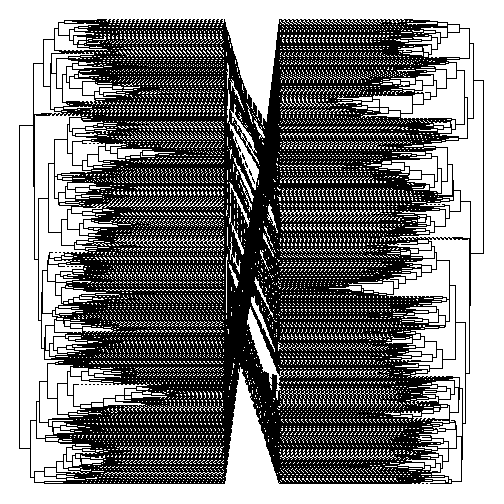``````## untangled "cophylo" plot
untangled<-cophylo(t1,t2,rotate=TRUE,print=TRUE)
``````
``````## Rotating nodes to optimize matching...
``````
``````## objective: 10141238
## objective: 10141238
## objective: 10141238
## objective: 10141238
## objective: 7131734
## objective: 7131734
## objective: 7131734
...
...
...
## objective: 0
## objective: 0
``````
``````## Done.
``````
``````plot(untangled,pts=FALSE,ftype="off")
``````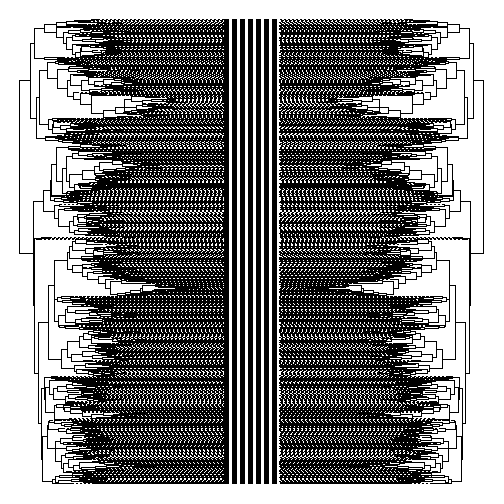(It may be hard to tell, but the value of our objective function, 0, tells us they match exactly!)

Finally, there is another case in which tips can be matched precisely - when they have the same “cladewise” order, but differ completely in topology. Interestingly, this is actually a problem that `cophylo` cannot solve:

``````t1<-rtree(n=80)
t2<-rtree(n=80)
t1\$tip.label<-t2\$tip.label<-paste("t",1:80,sep="")
obj<-cophylo(t1,t2,rotate=FALSE)
for(i in 1:200) t2<-rotateNodes(t2,sample(1:t2\$Nnode+Ntip(t2),1))
plot(obj,fsize=0.4)
``````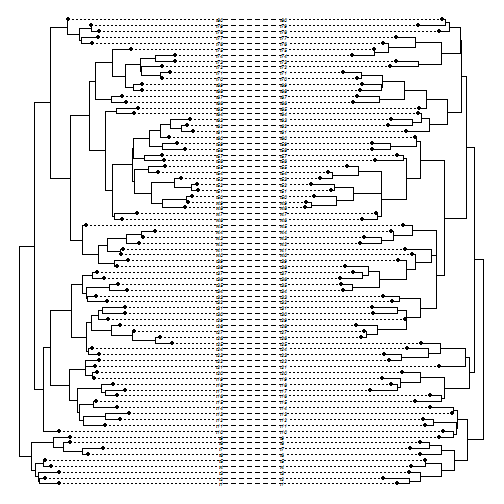``````obj<-cophylo(t1,t2,print=TRUE,methods=c("pre","post"))
``````
``````## Rotating nodes to optimize matching...
``````
``````## objective: 41422
## objective: 41422
...
...
...
## objective: 12256
``````
``````## Done.
``````
``````plot(obj,fsize=0.4)
``````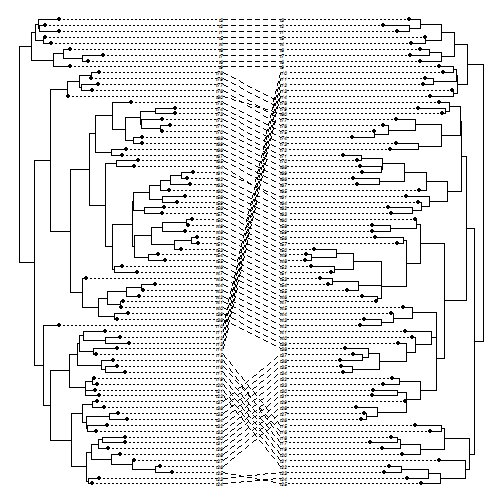That's all.